# Sign of y Axis LabelsHi,

I have standard y vs x plot. In my case the y values are negative. Therefore, the y axis labels are also negative. However, for presentation purposes only, I would like to change the y axis labels such that they are displayed displayed as positive numbers. My question is: Is there a way to obtain this effect without changing the values of the y-wave and without creating additional waves? In practice, I am able to obtain the effect I am looking for by multiplying the y-wave with -1 and then using the "swap" under the Axis Range tab to flip the y-curve to its original orientation. However, this procedure has the unfortunate effect that it changes the values of the original y-wave. Ideally, the solution I am looking for is a function which acts only on the y-labels and which can be implemented as necessary.

Thank you! & Happy New Year!

Hi Andy,

Thank you for your reply. If I understand correctly what you are suggesting is to use the command: ModifyGraph muloffset={0,-1} . However, when I tried this, it flipped the y curve but left the labels intact. Is there something I am missing?

Best,

Hi,

If the original wave had y values that were all negative, then the -1 multiplier would flip them to positive albeit in a mirror image.  The test data I had did show this effect and the corresponding tick labels also reflected the change in sign. Here is my example.  Note the Y axis is now showing an "unnatural" direction with increasing going down.  This retains the shape of the curve, though I would probably not reverse the axis myself.

Note: when I did the -1 multiplier the tick labels represented now positive numbers.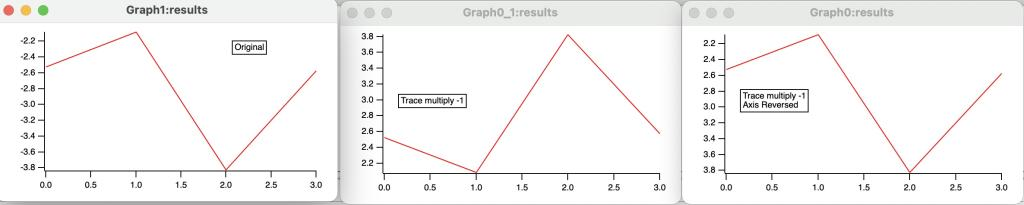Andy

Hi Andy,

Thank you again. I am sorry, but this is not quite what I would like to achieve. Specifically, I do not want to modify the values of the original  y-wave (because that would change many other things). For example, for a figure of a typical spectrum shown below, I am simply looking for a "cheap" way to remove the minus sign of the numerical values of the y-axis labels by simply modifying the labels. I was able to reproduce your example only by multiplying the original y-wave with "-1" which changes the values of the original data (which is analogous to the procedure I described in my original post which I would like to avoid).

Best,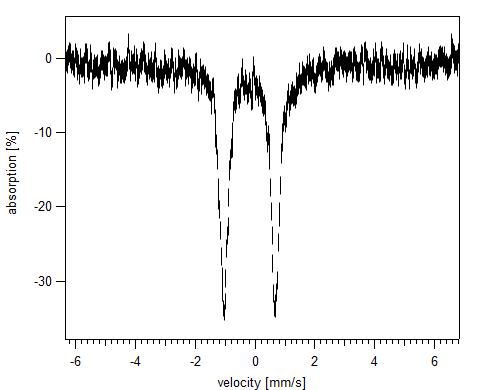Hi Andy,

It looks like I am missing something. When I followed your instructions this is what I obtained: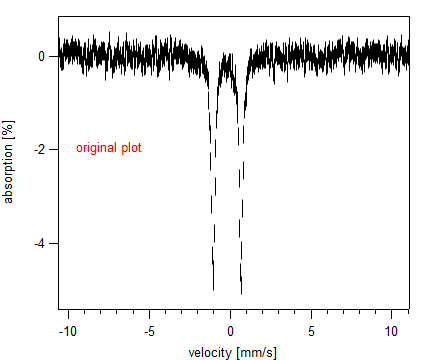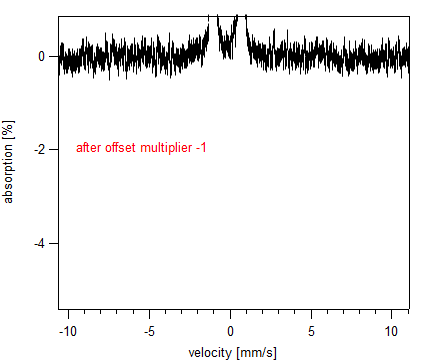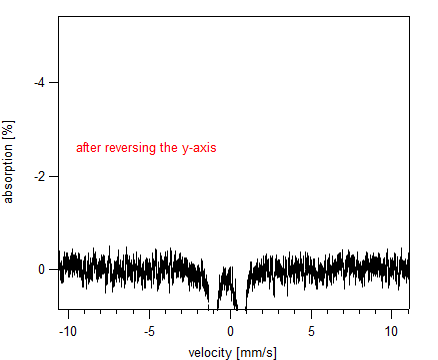It looks like at this stage, I need another step to change the limits of the y-axis by using the negative limits of the original plot's y-axis. I could get these limits using GetAxis.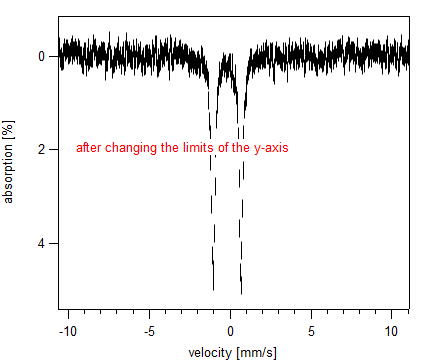Not quite what I expected but I think this should work.

Thank you!

just for the record here is the function which accomplishes my original goal following Andy's suggestions:

function remove_minus_of_y_axis_label()

string GraphName=WinName(0,1)
if(strlen(GraphName)==0)
return  0
endif

string traces=TraceNameList(GraphName,";",1)
variable i, n=ItemsInList(traces)

for(i=0;i<n;i++)
string traceName=StringFromList(i,traces)
ModifyGraph muloffset(\$traceName)={0,-1}
endfor

string y_axes_list=removefromList("bottom",axislist(GraphName))
n=ItemsInList(y_axes_list)

for(i=0;i<n;i++)
string axisname=stringfromList(i,y_axes_list)
GetAxis/Q \$axisname
variable y_min=-1*V_min, y_max=-1*V_max
setaxis \$axisname y_min, y_max
endfor

end function

Seem like you got the solution, but I would like to just mention that things do not need to get that complicated (and some other random notes which might be of help to you).

You could just call ModifyGraph muloffset={0,-1} once for the whole graph to reverse all traces at once.

It seem you have multiple y-axes with a manual range. In the case of autoscaling the axes everything would adjust automatically and you could call SetAxis/A/R axisName to reverse the axis.

Your code might fail if you have a 'top axis'. Change this if you want to make the function a bit more robust: y_axes_list=removefromList("top;bottom;",axislist(GraphName))

Also, function code in Igor is terminated with just 'end', not 'end function' (this is no error per se, however; any stuff after end is ignored).

I would like to offer a different solution, not necessarily better. It does involve creating two new waves, a label position wave and a label text wave.

First, bring up the Modify Axis dialog for the Y axis. On the Auto/Man Ticks tab, select User Ticks from Waves. In the User Tick Waves box, click the New From Auto Ticks button. Click Do It.

You now have a graph that looks exactly like it did before, but you have a couple of new waves. If you edit the new text wave to remove the minus signs, you will have achieved a lying axis!